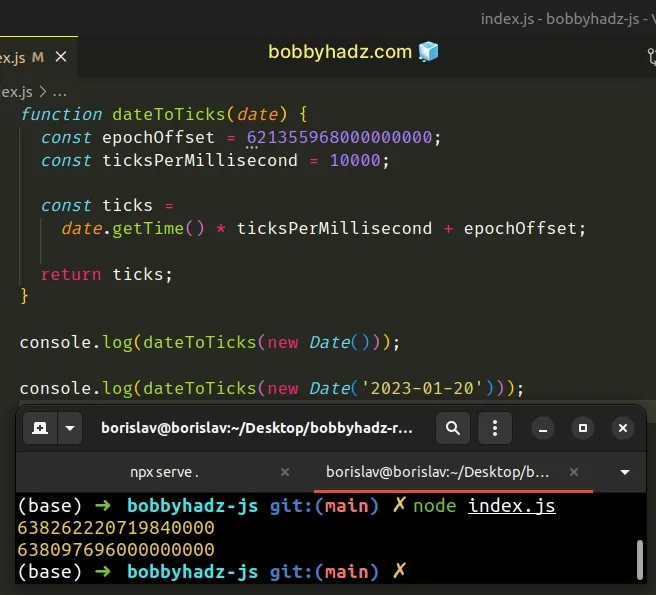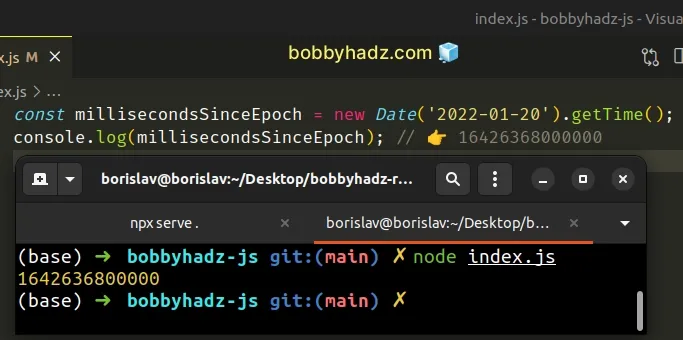# Convert a Date object to Ticks using JavaScriptLast updated: Jan 13, 2023
2 min## #Convert a Date object to Ticks using JavaScript

To convert a date object to ticks:

1. Use the `getTime()` method on the date to get the number of milliseconds since the Unix Epoch.
2. Multiply the timestamp by the number of ticks in a millisecond (10,000).
3. Add the epoch offset to the result.
index.js
```Copied!```function dateToTicks(date) {
const epochOffset = 621355968000000000;
const ticksPerMillisecond = 10000;

const ticks =
date.getTime() * ticksPerMillisecond + epochOffset;

return ticks;
}

console.log(dateToTicks(new Date()));

console.log(dateToTicks(new Date('2023-01-20')));
``````The `dateToTicks()` function takes a `Date` object as a parameter and converts it to universal ticks.

A single tick represents one hundred nanoseconds.

If you need the number of milliseconds elapsed between the 1st of January 1970 00:00:00 UTC and the given date, call the `getTime()` method on the `Date` object instead.
index.js
```Copied!```const millisecondsSinceEpoch = new Date('2022-01-20').getTime();
console.log(millisecondsSinceEpoch); // 👉️ 16426368000000
``````In our function, we stored the epoch offset in a variable. This is the number of nanoseconds between the 1st of January 0001 and the 1st of January 1970.

The second variable `ticksPerMillisecond` stores the number of .net ticks there are in a millisecond (10,000).

To get the total number of .net ticks, we have to multiply the milliseconds since the Unix Epoch by the number of ticks per millisecond and add the epoch offset.

The `getTime()` method returns the number of milliseconds since the Unix epoch, so multiplying the value by the number of ticks in `1` millisecond (10,000) gives us the number of ticks since Jan 1, 1970, 00:00:00 UTC.

Adding the epoch offset to the result gives us the total number of ticks since the 1st of January 0001.